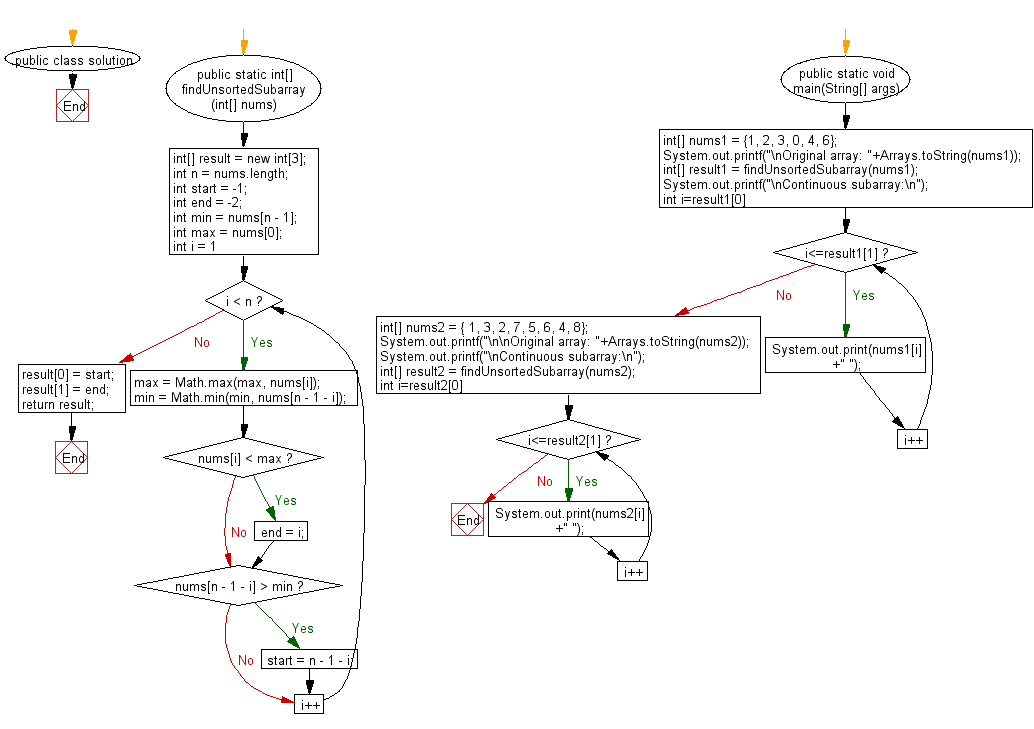﻿ Java: Find one continuous subarray to sort an entire array# Java Array Exercises: Find and print one continuous subarray to sort an entire array

## Java Array: Exercise-72 with Solution

Write a Java program to find and print one continuous subarray (from a given array of integers) that if you only sort the said subarray in ascending order then the entire array will be sorted in ascending order.

Example:
Input :
nums1 = {1, 2, 3, 0, 4, 6}
nums2 = { 1, 3, 2, 7, 5, 6, 4, 8}
Output:
Continuous subarray:
1 2 3 0
Continuous subarray:
3 2 7 5 6 4

Sample Solution:

Java Code:

``````import java.util.Arrays;
public class solution {
public static int[] findUnsortedSubarray(int[] nums) {
int[] result = new int;
int n = nums.length;
int start = -1;
int end = -2;
int min = nums[n - 1];
int max = nums;
for (int i = 1; i < n; i++) {
max = Math.max(max, nums[i]);
min = Math.min(min, nums[n - 1 - i]);
if (nums[i] < max) {
end = i;
}
if (nums[n - 1 - i] > min) {
start = n - 1 - i;
}
}
result = start;
result = end;

return result;
}

public static void main(String[] args)
{
int[] nums1 = {1, 2, 3, 0, 4, 6};
System.out.printf("\nOriginal array: "+Arrays.toString(nums1));

int[] result1 = findUnsortedSubarray(nums1);
System.out.printf("\nContinuous subarray:\n");
for(int i=result1; i<=result1; i++){
System.out.print(nums1[i] +" ");
}

int[] nums2 = { 1, 3, 2, 7, 5, 6, 4, 8};
System.out.printf("\n\nOriginal array: "+Arrays.toString(nums2));
System.out.printf("\nContinuous subarray:\n");
int[] result2 = findUnsortedSubarray(nums2);

for(int i=result2; i<=result2; i++){
System.out.print(nums2[i] +" ");
}
}
}
```
```

Sample Output:

```Original array: [1, 2, 3, 0, 4, 6]
Continuous subarray:
1 2 3 0

Original array: [1, 3, 2, 7, 5, 6, 4, 8]
Continuous subarray:
3 2 7 5 6 4
```

Flowchart:Java Code Editor:

Improve this sample solution and post your code through Disqus

What is the difficulty level of this exercise?

Test your Programming skills with w3resource's quiz.

﻿

## Java: Tips of the Day

countOccurrences

Counts the occurrences of a value in an array.

Use Arrays.stream().filter().count() to count total number of values that equals the specified value.

```public static long countOccurrences(int[] numbers, int value) {
return Arrays.stream(numbers)
.filter(number -> number == value)
.count();
}
```

Ref: https://bit.ly/3kCAgLb